GAM splines now easy in JAGS and OpenBUGS. An example on 2D spatial data

By | March 11, 2015

Last week I met Simon Wood, creator of `mgcv` package, which is THE tool for fitting Generalized Additive Models (GAM) in R.

Simon brought my attention to function `jagam` which he has just added to `mgcv`. The function allows to transform the ‘spline’ or ‘smooth’ component of GAM model formula into BUGS code, meaning that the flexibility of GAMs is now available for routine MCMC model fitting.

I often deal with geographically structured (spatially explicit) data, and so I am excited by the prospect of using `jagam` to build spatially explicit hierarchical models.

Why should spatial statisticians care about `jagam`?

Because fitting of spatial splines in JAGS and OpenBUGS has so far been a pain. Yet from my experience and from personal communication with others (e.g. C. Dormann, B. O’Hara, C. Beale, S. Wood), splines are a well-behaved way to model spatial autocorrelation that can be easily examined and visualized separatedly from the rest of the model.

Splines can also be a handy alternative to the popular Conditional Autoregressive Models (CAR) that are available in OpenBUGS, but unavailable in JAGS. Hence, the clarity and portability of JAGS is now available to spatial modellers.

The aim of this post

Here I will demonstrate the `jagam` function in action. I will fit a simple Poisson GAM (with X and Y coordinates as predictors) to spatially explicit count data. I will also check if I get the same expected values from `mgcv` and `JAGS`, given the same model.

These are the packages that I will need:

``````  library(mgcv)   # fits GAMs
library(spatstat) # the source of the example data
library(raster) # for operations with rasters
library(R2jags) # interface between R and JAGS``````

The data

I will use example dataset `bei` from `spatstat` package. The data are positions of 3605 individual trees of Beilschmiedia pendula (Lauraceae) in a 1000 by 500 metre rectangular sampling region in the tropical rainforest of Barro Colorado Island. The data are stored in a point process pattern `ppp` object.

Let’s plot the data:

``````  par(mai=c(0.5,0.3,0.3,0))
plot(bei, cex=0.1, main=NULL)``````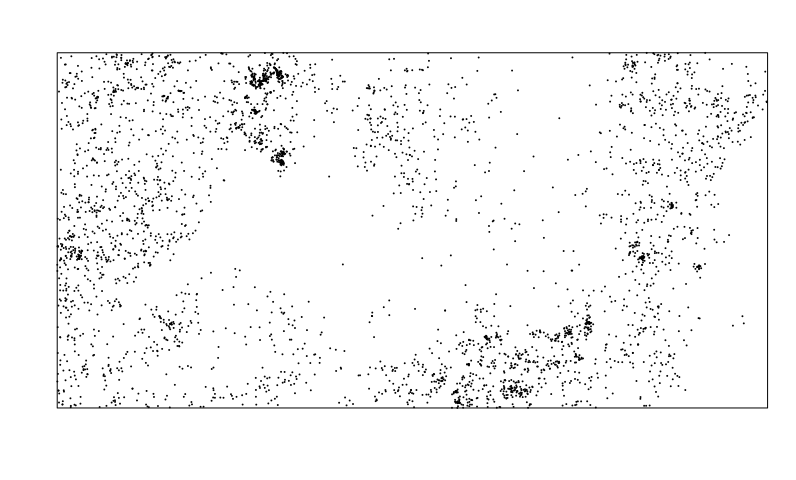I will fit the data into a raster of 25 x 50 grid cells; each grid cell gives the count of individual trees that fall within the cell:

``````  # cropping the data so that they have exactly 500 x 1000 cells
ext <- extent(0, 1000, 0, 500)         # spatial extent of the raster
empty <- raster(ext, nrow=25, ncol=50) # empty raster

# aggregating the point data into the raster
xy <- data.frame(x = bei\$x, y = bei\$y)
rst <- rasterize(xy, empty, fun = "count")

# replacing the NA values by 0
rst[is.na(rst)] <- 0

# extracting the cell values and their coordinates to a data.frame
coord <- xyFromCell(rst,1:ncell(rst))
count <- extract(rst, 1:ncell(rst))
all.data <- data.frame(coord, count=count)``````

This is the resulting rasterized dataset, with point locations of the trees plotted on top. The color gradient shows counts of trees in each grid cell.

``````  plot(rst, axes=FALSE)
points(xy, cex=0.1)``````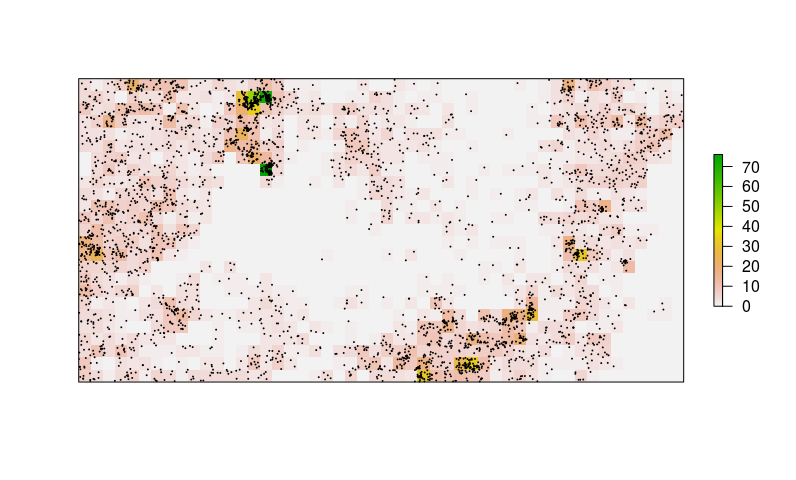Standard GAM in `mgcv`

This is the standard way to fit X- and Y- splines in `mgcv`:

``````  # the gam model with s() indicating that I fit splines
space.only <- gam(count~s(x, y), data=all.data, family = "poisson")
# extraction of the predictions
preds.mgcv <- as.vector(predict(space.only, type = "response"))

# putting the predictions into a raster
rst.mgcv <- rst
rst.mgcv[] <- preds.mgcv``````

This is the predicted surface on a map:

``````  plot(rst.mgcv, axes=FALSE)
points(xy, cex=0.1)``````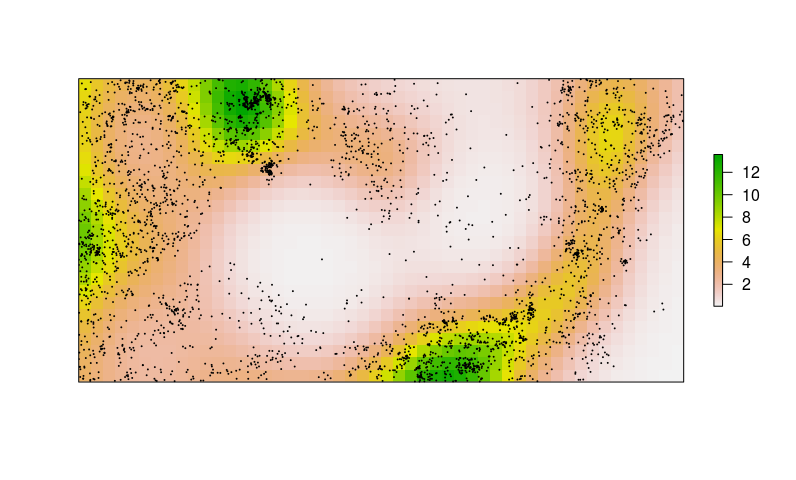The `jagam` function in action

The main point here is that the new `jagam` function takes the GAM formula and converts it into a piece of BUGS code. The resulting code can then be run in JAGS, or even in OpenBUGS. Here it is in action:

``````  jags.ready <- jagam(count~s(x, y),
data=all.data,
family="poisson",
file="jagam.bug")``````

The `jagam` function does two things: (1) it creates an object that contains the data in the list format that can be readily used in the `jags` function (package `R2jags`), and (2) it writes the BUGS model definition into a file. That makes it really easy to fit GAM splines in JAGS. The idea is that more complex hierarchical structures can then be added directly into the BUGS code.

Let’s have a look into the `jagam.bug` file:

``  readLines("jagam.bug")``
``````##   "model {"
##   "  eta <- X %*% b ## linear predictor"
##   "  for (i in 1:n) { mu[i] <-  exp(eta[i]) } ## expected response"
##   "  for (i in 1:n) { y[i] ~ dpois(mu[i]) } ## response "
##   "  ## Parameteric effect priors CHECK tau is appropriate!"
##   "  for (i in 1:1) { b[i] ~ dnorm(0,0.001) }"
##   "  ## prior for s(x,y)... "
##   "  K1 <- S1[1:29,1:29] * lambda  + S1[1:29,30:58] * lambda"
##   "  b[2:30] ~ dmnorm(zero[2:30],K1) "
##  "  ## smoothing parameter priors CHECK..."
##  "  for (i in 1:2) {"
##  "    lambda[i] ~ dgamma(.05,.005)"
##  "    rho[i] <- log(lambda[i])"
##  "  }"
##  "}"``````

Fitting the model in JAGS

Here I fit the Bayesian model by calling `jags` function from package `R2jags`. I will monitor the expected values (`mu`) in each grid cell of the raster.

``````  model.fit <- jags(data=jags.ready\$jags.data,
model.file="jagam.bug",
parameters.to.save=c("mu"),
n.chains=3,
n.iter=1000,
n.burnin=500)``````
``## module glm loaded``
``````## Compiling model graph
##    Resolving undeclared variables
##    Allocating nodes
##    Graph Size: 42982
##
## Initializing model``````
``````  # extracting the fitted means
preds.mu <- as.vector(model.fit\$BUGSoutput\$mean\$mu)

# inserting the fitted means to the raster
rst.jags <- rst
rst.jags[] <- preds.mu``````

Let’s plot the JAGS results:

``````  plot(rst.jags, axes=FALSE)
points(xy, cex=0.1) # adding the positions of individual trees``````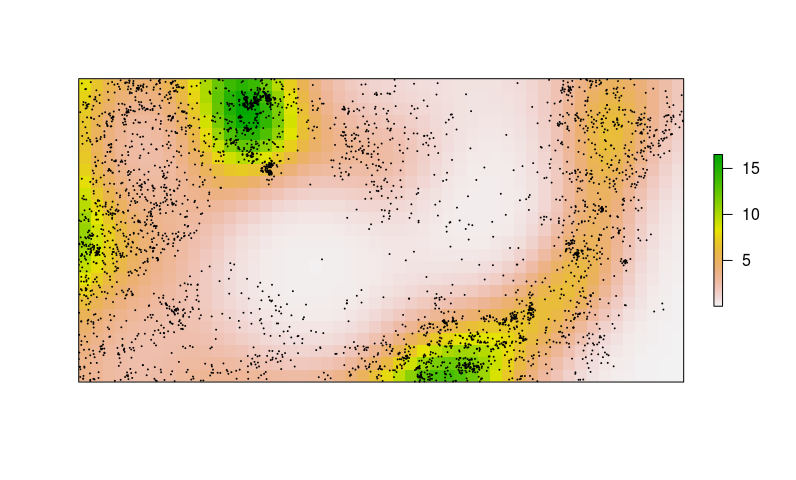It looks almost identical to the `mgcv` output. Let’s have a closer look.

`mgcv` vs JAGS

Here I compare the modelled counts from the two models.

``````  plot(preds.mgcv, preds.mu,
xlab="Counts from mgcv",
ylab="Counts from JAGS")
abline(a=0, b=1, col="red", lwd=2)``````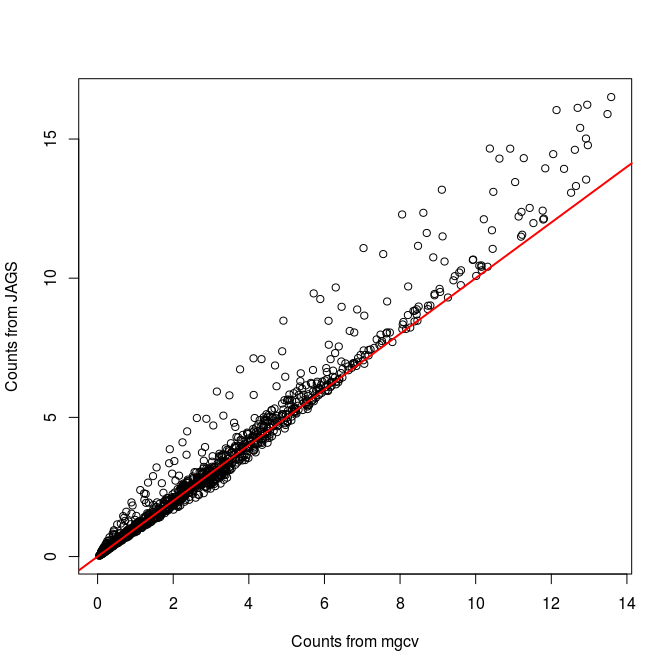The predicted counts are not identical, JAGS predicts relatively higher counts than `mgcv`. I am not sure if this is important – maybe it has something to do with my priors, I don’t know. But I guess that I don’t care that much, as long as the predictions are roughly similar.

(Visited 4,654 times, 2 visits today)

10 thoughts on “GAM splines now easy in JAGS and OpenBUGS. An example on 2D spatial data”

1.Ally

This is really cool! Thanks a lot . Ally

2.Marc Kery

Hi Petr,

this is great, thanks. One thing though: for running JAGS from R I would suggest to use the new jagsUI package, which is better than R2jags, though it has almost the same format as R2jags, R2WinBUGS and R2OpenBUGS.

Regards -- Marc

1.Petr Keil Post author

Hi Marc, thanks for the tip! I guess I should try jagsUI the next time I am running MCMC, it looks promising.

3.Paul Lantos

This is fantastic!

If I'm running a binomial/logit spatial GAM like this, how could I make predictions from the Jags output? Normally I would like to take the GAM output and predict log-odds (or odds ratio vs a non-spatial model) onto an x-y grid that covers the spatial area. Not sure how to do this from the Jags output.

4.Ice

Thanks. When I use ns() function from Splines Package in jagam() function, there is no spline term in the BUGS model. Could anyone tell me how to realize it? I just want my model be the same as that when I use gam() function.
Like:
jagam(y ~ x1 + ns(x2, df=7), family=possion)

1.Petr Keil Post author

Hi,
The help to the jagam() function states that you can use "s", "te", "ti" or "t2" splines. So that is probably why it does not work with "ns" splines.
One solution would be not to use JAGS, but STAN for that. There is a packages "brms" which allows you to fit GAMs with any kinds of splines in the Bayesian setting. It's great!
Cheers, Petr

5.LilianChief

I have checked your site and i have found some duplicate content, that's why you don't rank high
6.Allan